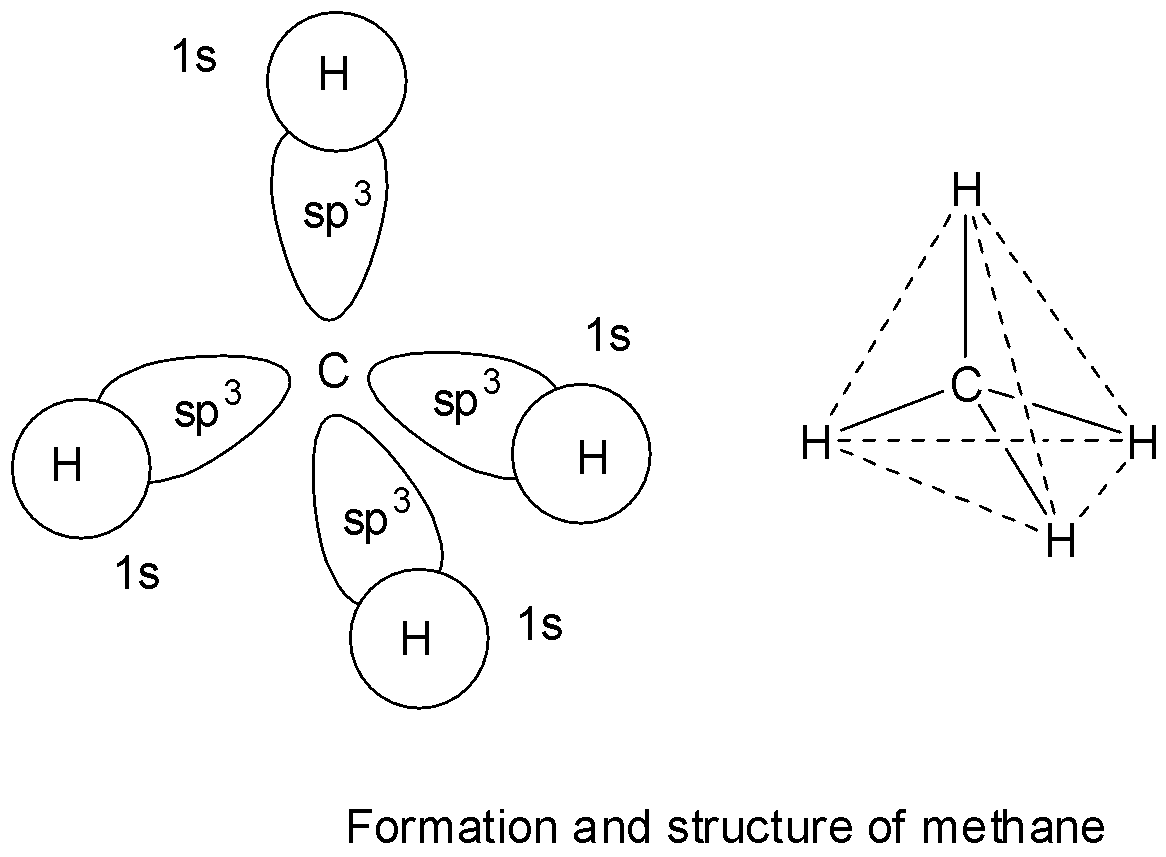QuestionAnswers

# The hybridization on the central carbon in methane, ${\text{C}}{{\text{H}}_{\text{4}}}$ , is:(A) ${\text{sp}}$ (B) ${\text{s}}{{\text{p}}^2}$(C) ${\text{s}}{{\text{p}}^3}$(D) ${\text{s}}{{\text{p}}^3}{\text{d}}$

Hint: The term hybridization is used to refer to the intermixing of atomic orbitals having nearly the same energies which result in their rearrangement to form completely different orbitals which are called hybrid orbitals. The hybridization and bond formation of carbon occurs in three ways: ${\text{s}}{{\text{p}}^3}$ , ${\text{s}}{{\text{p}}^2}$ and ${\text{sp}}$ hybridization.

In methane, carbon has 4 valence electrons and takes 4 more electrons to complete its octet. The four half filled orbitals of carbon in methane before getting involved in bond formation, mix up their energies and rearrange and redistribute equally to give four new and equivalent ${\text{s}}{{\text{p}}^3}$ hybrid orbitals which are directed at an angle of ${109^\circ }28'$ with respect to one another. These 4 ${\text{s}}{{\text{p}}^3}$ hybrid orbitals of carbon then overlap with 1s orbitals of 4 hydrogen atoms to give four ${\text{s - s}}{{\text{p}}^3}$ sigma bonds. The four bonds in methane are directed towards the 4 corners of a tetrahedron.

So, option C is correct.* In ${\text{sp}}$ hybridization, the carbon atom in excited state is involved in the hybridization of its 2s orbital with one 2p orbital while the other two 2p orbitals are left unchanged. As a result, two new ${\text{sp}}$ hybrid orbitals form making an angle of ${180^\circ }$ with each other. The central carbon atom has linear shape. So, option A is wrong.
* In ${\text{s}}{{\text{p}}^2}$ hybridization, the carbon atom in excited state is involved in the hybridization of its 2s orbital with two 2p orbitals while the third 2p orbital is left unchanged. As a result, three new ${\text{s}}{{\text{p}}^2}$ hybrid orbitals are formed making an angle of ${120^\circ }$ with each other. So, option B is wrong.
Carbon cannot show ${\text{s}}{{\text{p}}^3}{\text{d}}$ hybridization as it is a p-block element and has no d-orbitals. So, option D is also wrong.

Note: The characteristics of hybridization are:
* The number of hybrid orbital that are formed are equal to that of the hybridizing orbitals.
* All the hybrid orbitals have equivalent energy and shape.
* Hybrid orbitals taking part in bond formation must have one electron in it.
* Electronic repulsions between the hybrid orbitals make them stay at the maximum distance apart.